# Estimating microclimates

#### 2023-09-13

# Load TrenchR package

library(TrenchR)

This tutorial covers approaches and functions for preparing environmental data for analyses such as examining organismal energy balances. The tutorial first addresses estimating the intensity and partitioning of solar radiation, including estimating solar angles and day length. We then turn to estimating temperature and wind speed profiles that can scale weather station data to the conditions experienced by organisms. We review functions for modeling diurnal variation in temperature and solar radiation as well as heat accumulation (degree days). The tutorial concludes with a microclimate model for estimating soil temperatures. Additional resources are available in R packages including NicheMapR, microclima, and microclimc. Functions are generally from Campbell and Norman (2012) unless other resources are specified. More detailed source information is available in each function.

## Solar angles and day length

Sun position and day length are often estimated based on the calendar date. We provide a function day_of_year() that facilitates translating a date into a calendar date.

day_of_year(day    = "2017-04-22",
format = "%Y-%m-%d")
##  112

We next present functions to calculate sun angles. We use the calendar date (J) to estimate solar declination (radian), which is the angular distance of the sun north or south of the earth’s equator, as follows (dec_angle()):

$\delta= \arcsin[0.39795 \cos(0.21631+ 2 \arctan (0.967 \tan [0.0086(-186+J)]))].$

The solar declination angle can be calculated in TrenchR as follows:

plot(x    = 1:365,
y    = dec_angle(1:365),
type = "l",
xlab = "day of year",
ylab = "declination angle (radian)")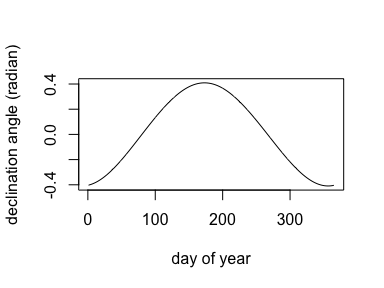Zenith angle $$\psi$$ (°), the location of the sun as an angle measured from vertical, can be estimated as:

$\cos \psi = \sin( \delta) \sin( \phi) + \cos(\delta)\cos(\phi)\cos(\pi/12*(h-h_0)),$

where $$J$$ is calendar date, $$\phi$$ is latitude (radians), $$h$$ is hour, and $$h_0$$ is the time of solar noon. We illustrate how zenith angles (°) change over the day throughout the year in Seattle, WA, USA:

zenith <- zenith_angle(hour = 1:24,
doy  = 200,
lat  = 47.61,
lon  = -122.33)

plot(x    = 1:24,
y    = zenith,
type = "l",
ylab = "zenith angle (°)",
xlab = "hour")

zenith <- zenith_angle(hour = 1:24,
doy  = 1,
lat  = 47.61,
lon  = -122.33)
points(x    = 1:24,
y    = zenith,
type = "l",
lty  = "dotted")

zenith <- zenith_angle(hour = 1:24,
doy  = 100,
lat  = 47.61,
lon  = -122.33)
points(x    = 1:24,
y    = zenith,
type = "l",
lty  = "dotdash")

zenith <- zenith_angle(hour = 1:24,
doy  = 300,
lat  = 47.61,
lon  = -122.33)
points(x    = 1:24,
y    = zenith,
type = "l",
lty  = "dashed")

legend(x      = "bottomleft",
title  = "day of year",
legend = c(1, 100, 200, 300),
lty    = c("dotted", "dotdash", "solid", "dashed"))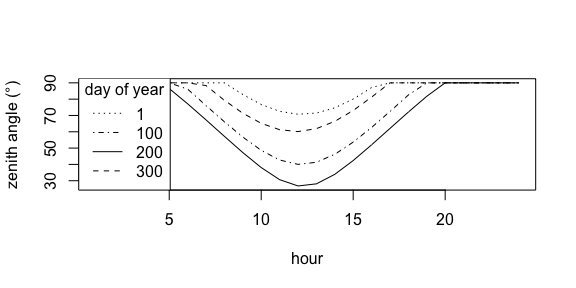We also examine how zenith angles (°) at noon change over the course of the year for latitudes along the longitude of Seattle, WA USA:

zenith <- zenith_angle(doy  = 1:365,
hour = 12,
lat  = 60,
lon  = -122.33)

plot(x    = 1:365,
y    = zenith,
type = "l",
ylim = range(0,90),
ylab = "zenith angle (°)",
xlab = "day of year")

zenith <- zenith_angle(doy  = 1:365,
hour = 12,
lat  = 40,
lon  = -122.33)
points(x    = 1:365,
y    = zenith,
type = "l",
lty  = "dotted")

zenith <- zenith_angle(doy  = 1:365,
hour = 12,
lat  = 20,
lon  = -122.33)

points(x    = 1:365,
y    = zenith,
type = "l",
lty  = "dotdash")

zenith <- zenith_angle(doy  = 1:365,
hour = 12,
lat  = 0,
lon  = -122.33)

points(x    = 1:365,
y    = zenith,
type = "l",
lty  = "dashed")

legend(x      = "topleft",
title  = "latitude (°)",
legend = c(0, 20, 40, 60),
lty    = c("dashed", "dotdash", "dotted", "solid"))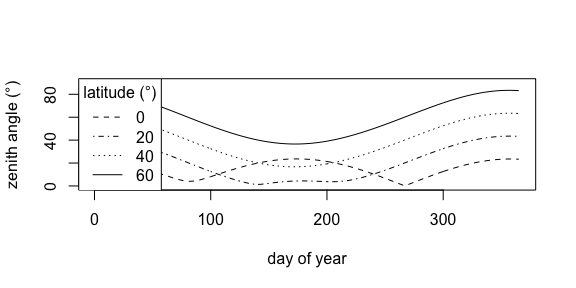The time of solar noon (solar_noon()) can be estimated as $$h_0= 12 - LC -ET$$, where $$LC$$ in the longitude correction (+4 minutes for each degree east of the standard meridian and -4 minutes for each degree west of the standard meridian) and $$ET$$ is the equation of time. The $$ET$$ is estimated as: $ET= \frac{-104.7\sin (f)+596.2\sin (2f)+4.3\sin (3f)-12.7\sin (4f)-429.3\cos (f)-2.0\cos (2f)+19.3\cos (3f)}{3600},$where $$f=279.575 + 0.9856 J$$, in degrees. The time of solar noon can be estimated across the year for several longitudes as follows:

plot(x    = 1:365,
y    = solar_noon(lon = 150, doy = 1:365),
type = "l",
ylim = c(11.3,12.7),
ylab = "time of solar noon (hour)",
xlab = "day of year")

points(x    = 1:365,
y    = solar_noon(lon = 155, doy = 1:365),
type = "l",
lty  = "dotted")

points(x    = 1:365,
y    = solar_noon(lon = 145, doy = 1:365),
type = "l",
lty  = "dashed")

abline(a = 12,
b = 0)

legend(x      = "topright",
title  = "longitude (°)",
legend = c(145, 150, 155),
lty    = c("dashed", "solid", "dotted"))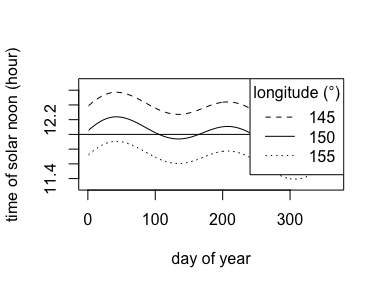The azimuth angle is the angle (°) from which the sunlight is coming measured from true north or south measured in the horizontal plane. The azimuth angle ($$AZ$$, °) is estimated with respect to due south, increasing in the counter-clockwise direction such that $$90^\circ$$ is east. $$AZ$$ can be calculated as a function of declination angle $$\delta$$ (°), zenith angle $$\psi$$ (°), and latitude $$\phi$$ (°) as follows:

$\cos AZ= \frac{-(\sin \delta - \cos \psi \sin \phi)}{\cos \phi \sin \psi}.$

TrenchR includes a function to estimate azimuth angle as follows. We first examine how azimuth angle varies across the day:

az <- unlist(lapply(5:18,
FUN = azimuth_angle,
doy = 173,
lat = 47.61,
lon = -122.33))

plot(x    = 5:18,
y    = az,
type = "l",
xlab = "hour",
ylab = "azimuth angle (°)")

az <- unlist(lapply(5:18,
FUN = azimuth_angle,
doy = 356,
lat = 47.61,
lon = -122.33))

points(x    = 5:18,
y    = az,
type = "l",
lty  = "dashed")

az <- unlist(lapply(5:18,
FUN = azimuth_angle,
doy = 266,
lat = 47.61,
lon = -122.33))

points(x    = 5:18,
y    = az,
type = "l",
lty  = "dotted")

az <- unlist(lapply(5:18,
FUN = azimuth_angle,
doy = 228,
lat = 47.61,
lon = -122.33))

points(x    = 5:18,
y    = az,
type = "l",
lty  = "dotdash")

legend(x      = "topleft",
title  = "day of year",
legend = c(173, 228, 266, 356),
lty    = c("solid", "dotdash", "dotted", "dashed"))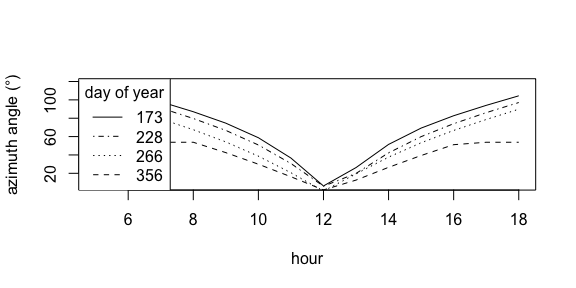We then examine how azimuth angle varies across the year:

az <- unlist(lapply(1:365,
FUN = azimuth_angle,
hour = 12,
lat = 47.61,
lon = -122.33))

plot(x    = 1:365,
y    = az,
type = "l",
xlab = "day of year",
ylab = "azimuth angle (°)", ylim=c(0,120))

az <- unlist(lapply(1:365,
FUN = azimuth_angle,
hour = 9,
lat = 47.61,
lon = -122.33))

points(x    = 1:365,
y    = az,
type = "l",
lty  = "dashed")

az <- unlist(lapply(1:365,
FUN = azimuth_angle,
hour = 6,
lat = 47.61,
lon = -122.33))

points(x    = 1:365,
y    = az,
type = "l",
lty  = "dotted")

legend(x      = "topright",
title  = "hour",
legend = c(6, 9, 12),
lty    = c("dotted", "dashed", "solid"))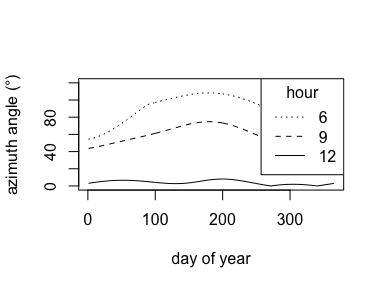These sun angles can be used to solve for day length. The equation for half day length $$h_s$$, which is the time (°) from sunrise to solar noon, is used as the basis for our daylength() function:

$h_s= \cos^{-1}\left( \frac{\cos \psi-sin \phi \sin \delta}{\cos \phi \cos \delta} \right).$

We examine how day length varies over the year for sites at several latitudes.

plot(x    = 1:365,
y    = daylength(lat = 10, doy = 1:365),
type = "l",
ylim = c(8, 22),
xlab = "day of year",
ylab = "day length (hour)")

points(x    = 1:365,
y    = daylength(lat = 35, doy = 1:365),
type = "l",
lty  = "dashed")

points(x    = 1:365,
y    = daylength(lat = 60, doy = 1:365),
type = "l",
lty   = "dotted")

legend(x      = "topright",
title  = "latitude (°)",
legend = c(10, 35, 60),
lty    = c("solid", "dashed", "dotted"))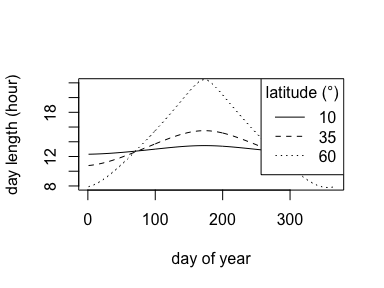We provide functions to estimate solar (shortwave) radiation by discounting incoming solar radiation due to scattering as the radiation travels through the atmosphere. The primary function solar_radiation() returns estimates of the direct, diffuse, and reflected components of solar radiation ($$W m^{-2}$$). The radiation streams are the direct irradiance on a surface perpendicular to the beam $$S_p$$, the diffuse sky irradiance on a horizontal plane $$S_d$$ ($$W m^{-2}$$), and the reflected radiation from the ground $$S_r$$. The sum of these three streams represents the total irradiance of a horizontal surface $$S_t$$.

Calculation of these flux densities requires introducing several additional quantities. The atmospheric transmissivity, $$\tau$$, ranges between 0.6 and 0.7 for typical clear sky conditions (Gates 2012). The solar constant, $$S_{p0}$$, approximates extraterrestrial flux density as $$1,360 W m^{−2}$$. The optical air mass number, $$m_a$$, is the ratio of slant path length through the atmosphere to zenith path length and is a function of atmospheric pressure: $$m_a=p_a/(101.3\cos(\psi))$$, where $$p_a$$ is air pressure (kPa) and $$\psi$$ is zenith angle (°, see above). The function airpressure_from_elev() estimates air pressure as $$p_a (kPa)= 101.3 e ^{\frac{-E}{8200}}$$, where $$E$$ is the elevation in meters above sea level. TrenchR provides a function to estimate air pressure from elevation (m).

plot(x    = 1:4000,
y    = airpressure_from_elev(elev = 1:4000),
type = "l",
xlab = "elevation (m)",
ylab = "air pressure (kPa)")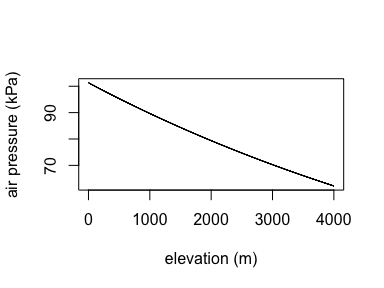Direct irradiance is a function of the distance a solar beam travels through the atmosphere; the transmittance of the atmosphere, $$\tau$$; and the incident flux density, $$S_{p0}$$: $$S_p=S_{p0}\tau^{m_a}$$. We approximate diffuse radiation using an empirical relation (Liu and Jordan 1960), $$S_d=0.3(1-\tau^{m_a}) S_{p0}\cos\psi$$. Finally, reflected radiation is the product of albedo, $$\rho_S$$, and the sum of direct and diffuse radiation: $$S_r= \rho_S (S_p+S_d)$$. We estimate the direct, diffuse, and reflected components of solar radiation ($$W m^{-2}$$) as follows.

We estimate how solar radiation components vary diurnally.

oldpar<-par()

par(mar=c(5, 5, 3, 2))

zen <- unlist(lapply(1:24,
FUN = zenith_angle,
doy = 200,
lat = 47.61,
lon = -122.33))

zen <- zen * 2 * pi / 360

rd <- lapply(zen,
doy  = 200,
tau  = 0.6,
elev = 1500,
rho  = 0.7)

rd <- matrix(unlist(rd),
nrow  = 3,
byrow = FALSE)

plot(x    = 1:24,
y    = rd[1,],
type = "l",
xlab = "hour",
ylim = c(0, 800))

points(x    = 1:24,
y    = rd[2,],
type = "l",
lty = "dotted")

points(x    = 1:24,
y    = rd[3,],
type = "l",
lty  = "dashed")

legend(x      = "topright",
legend = c("direct", "diffuse", "reflected"),
lty    = c("solid", "dotted", "dashed"))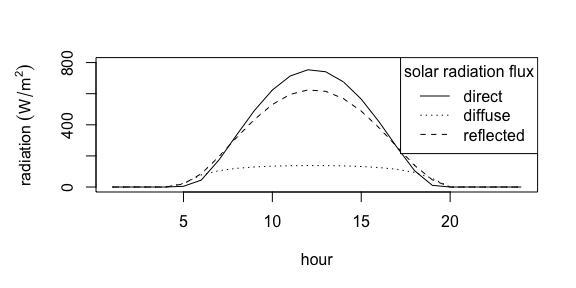We then examine how solar radiation components vary as a function of zenith angle.

par(mar = c(5, 5, 3, 2))

psi.seq <- seq(from = 0,
to   = 90,
by   = 5)

rd <- lapply(psi.seq * 2 * pi / 360,
doy  = 200,
tau  = 0.75,
elev = 1500,
rho  = 0.7)

rd <- matrix(unlist(rd),
nrow  = 3,
byrow = FALSE)

plot(x    = psi.seq,
y    = rd[1,],
type = "l",
xlab = "zenith angle (°)",
ylim = c(0, 1200))

points(x    = psi.seq,
y    = rd[2,],
type = "l",
lty = "dotted")

points(x    = psi.seq,
y    = rd[3,],
type = "l",
lty  = "dashed")

legend(x      = "topright",
legend = c("direct", "diffuse", "reflected"),
lty    = c("solid", "dotted", "dashed"))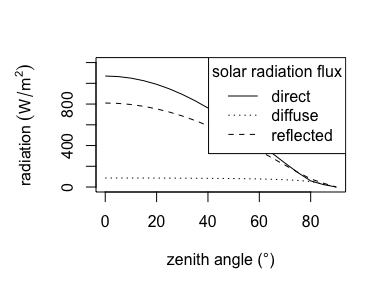Similar but alternative algorithms for estimating hourly direct solar radiation ($$W m^{-2}$$) were adapted from a comparison of the methods by Tracy et al. (1983). Algorithms from earlier versions of Campbell and Norman (2012) and Gates (2012) are implemented. Both estimate radiation as a function of latitude, day of year, elevation, and time.

par(mar = c(5, 5, 3, 2))

r.seq <- lapply(seq(4, 20),
lat    = 43.57,
doy    = 112,
elev   = 866,
t0     = 12,
method = "Campbell 1977")

r.seq <- unlist(r.seq)

plot(x    = seq(4, 20),
y    = r.seq,
type = "l",
xlab = "hour",

r.seq <- lapply(seq(4, 20),
lat    = 43.57,
doy    = 112,
elev   = 866,
t0     = 12,
method = "Gates 1962")

points(x    = seq(4, 20),
y    = r.seq,
type = "l",
lty  = "dashed")

legend(x      = "topright",
legend = c("Campbell 1977", "Gates 1962"),
lty    = c("solid", "dashed"))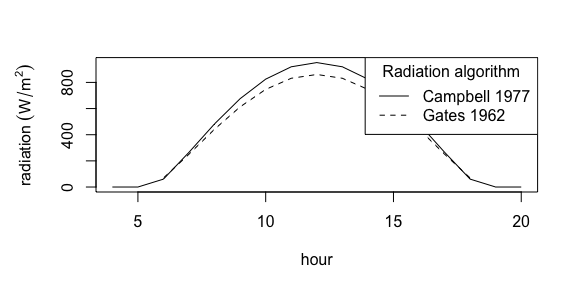We additionally provide a function (monthly_solar_radiation()) implementing an algorithm to estimate average monthly solar radiation ($$W m^{-2} day^{-1}$$) using basic topographic and climatic information as input. Cloudiness is stochastically modeled, so output will vary between functional calls and the relationship in the plot deviates from a smooth curve.

par(mar = c(5, 5, 3, 2))

r.seq <- lapply(seq(1, 365, 31),
lat  = 43.57,
lon  = -116.22,
elev = 866,
T    = 20,
hp   = 15,
P    = 15)

r.seq <- unlist(r.seq)

plot(x    = seq(1, 365, 31),
y    = r.seq,
type = "l",
xlab = "day of year",
ylab = expression(radiation ~ (W/m^{2})))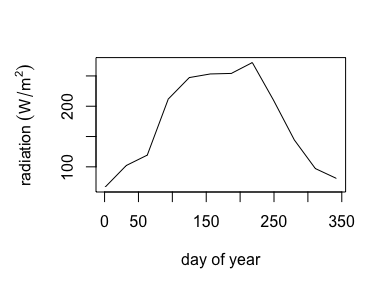We provide a function partition_solar_radiation() to estimate the diffuse fraction $$k_d$$ that can be used to partition measures of solar radiation into direct and diffuse components. The partitioning is based on empirically-derived relations estimated as a function of the clearness index $$k_t$$, which is the ratio of the global solar radiation measured at the surface to the total solar radiation at the top of the atmosphere. The relations are compiled in Wong and Chow (2001).

par(mar=c(5, 5, 3, 2))

r.seq <- lapply(seq(0, 1, 0.1),
method   = "Erbs",
lat      = 40,
sol.elev = 60)

plot(x    = seq(0, 1, 0.1),
y    = r.seq,
type = "l",
xlab = expression("clearness index" ~ k[t]),
ylab = "diffuse fraction",
ylim = c(0, 1))

r.seq <- lapply(seq(0, 1, 0.1),
method   = "Liu_Jordan",
lat      = 40,
sol.elev = 60)

points(x    = seq(0, 1, 0.1),
y    = r.seq,
type = "l",
lty  = "dashed")

r.seq <- lapply(seq(0, 1, 0.1),
method   = "Orgill_Hollands",
lat      = 40,
sol.elev = 60)

points(x    = seq(0, 1, 0.1),
y    = r.seq,
type = "l",
lty  = "dotted")

r.seq <- lapply(seq(0, 1, 0.1),
method   = "Olyphant",
lat      = 40,
sol.elev = 60)

points(x    = seq(0, 1, 0.1),
y    = r.seq,
type = "l",
lty  = "dotdash")

r.seq <- lapply(seq(0, 1, 0.1),
method   = "Reindl-1",
lat      = 40,
sol.elev = 60)

points(x    = seq(0, 1, 0.1),
y    = r.seq,
type = "l",
lty  = "longdash")

r.seq <- lapply(seq(0, 1, 0.1),
method   = "Reindl-2",
lat      = 40,
sol.elev = 60)

points(x    = seq(0, 1, 0.1),
y    = r.seq,
type = "l",
lty  = "longdash")

r.seq <- lapply(seq(0, 1, 0.1),
method   = "Lam_Li",
lat      = 40,
sol.elev = 60)

points(x    = seq(0, 1, 0.1),
y    = r.seq,
type = "l",
lty  = "twodash")

legend(x      = "topright",
title  = "Partitioning method",
legend = c("Erbs", "Liu_Jordan", "Orgill_Hollands", "Olyphant", "Reindl-1", "Reindl-2", "Lam_Li"),
lty    = c("solid", "dashed", "dotted", "dotdash", "longdash", "longdash", "twodash"))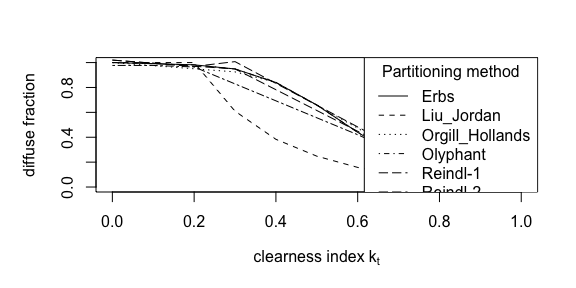The functions above are fairly coarse approximations. The SOLRAD routine by McCullough and Porter (1971) accounts for additional determinations of the diffuse fractions such as zenith angle, atmospheric pressure, and surface albedo. The SOLRAD routine is implemented in NicheMapR. TrenchR implements a numeric approximation to the SOLRAD routine from Tracy et al. (1983). We illustrate how the diffuse proportion shifts as a function of zenith angle for several different levels of atmospheric pressure and note the function general estimates a lower fraction of diffuse radiation than the algorithms above.

par(mar = c(5, 5, 3, 2))
r.seq <- lapply(seq(20, 85),
p_a = 86.1,
rho = 0.25)

plot(x    = seq(20,85),
y    = r.seq,
type = "l",
xlab = "zenith angle (°)",
ylab = "diffuse fraction")

r.seq <- lapply(seq(20, 85),
p_a = 96.1,
rho = 0.25)

points(x    = seq(20,85),
y    = r.seq,
type = "l",
lty  = "dashed")

r.seq <- lapply(seq(20, 85),
p_a = 76.1,
rho = 0.25)

points(x    = seq(20,85),
y    = r.seq,
type = "l",
lty = "dotted")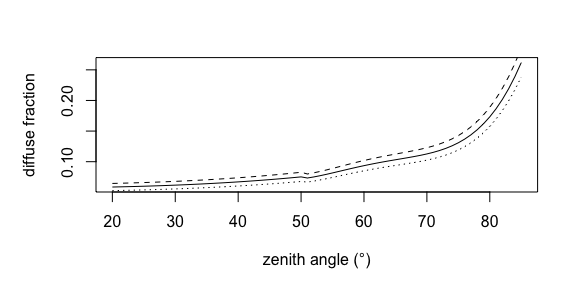# Temperature and wind speed profiles

Temperatures and wind speeds, standardly collected at a height of 1-2 m, can differ dramatically from conditions near the ground where most organisms live. Turbulent airflow influenced by the ground surface roughness sets up temperature and wind speed profiles. These profiles can be estimated to scale temperatures and wind speeds to organism height. We scale across height by estimating a wind speed profile using the relationship:

$u(z)= \frac{u^*}{0.41}ln \frac{z-d}{z_m}$

where $$u(z)$$ is the wind speed ($$m s^{-1}$$) at height $$z$$ (m), $$u^*$$ is the friction velocity ($$m s^{-1}$$), $$d$$ is the zero-plane displacement (m, the height at which wind speed is zero), and the constant 0.41 is the von Karman constant.

We provide a function to use this relationship to estimate surface roughness from wind speed measurements at a vector of heights (surface_roughness()). First, the zero-plane displacement is estimated as the intercept of the relationship between the wind velocities and the log of measurement height. Surface roughness $$z_0$$ can then be estimated by repeating the regression accounting for the zero-plane displacement (by subtracting the zero-plane displacement from the measurement heights). $$z_0$$ is estimated as the exponential of the ratio of the intercept and slope of the regression relationship as follows.

We illustrate estimating surface roughness.

u_r <- c(0.01, 0.025, 0.05, 0.1, 0.2)
zr  <- c(0.1, 0.25, 0.5, 0.75, 1)

surface_roughness(u_r = u_r,
zr  = zr)
##  0.06485225

We use the following relationship to estimate air temperature and wind speed at height $$z$$ under neutral conditions (no buoyancy associated with differential density):

$\frac{u_z}{u_r}=\frac{ln(z/z_0+1)}{ln(z_r/z_0+1)}=\frac{T_z-T_0}{T_r-T_0}$

where $$u_z$$ and $$u_r$$ are the wind speeds ($$m s^{-1}$$) at height $$z$$ (m) and reference height $$r$$ (m), respectively. $$T_z$$, $$T_r$$, and $$T_0$$ are air and soil temperatures at heights $$z$$ and $$r$$ and soil surface temperature, respectively. We provide this relationship in the wind_speed_profile_neutral() function, which estimates wind speed at a specified height. We additionally provide this relationship in the air_temp_profile_neutral() function, which estimates temperature at a specified height.

We examine how the wind speed profiles varies with wind speed (m/s).

zs <- seq(0, 2, 0.1)

us <- wind_speed_profile_neutral(u_r = 1,
zr  = 2,
z0  = 0.2,
z   = zs)

plot(x    = us,
y    = zs,
type = "l",
xlab = "wind speed, u (m/s)",
ylab = "height, z (m)")

points(x    = wind_speed_profile_neutral(u_r = 0.5,
zr  = 2,
z0  = 0.2,
z   = zs),
y    = zs,
type = "l",
lty  = "dashed")

points(x    = wind_speed_profile_neutral(u_r = 0.25,
zr  = 2,
z0  = 0.2,
z   = zs),
y    = zs,
type = "l",
lty  = "dotted")

legend(x      = "bottomright",
title  = "wind speed at 2m",
legend = c(0.25, 5, 1),
lty    = c("dotted", "dashed", "solid"))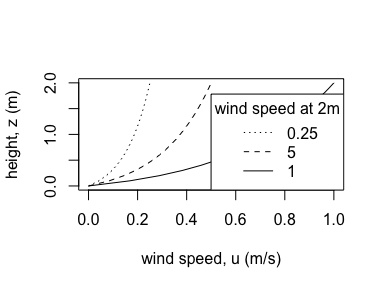We next illustrate the influence of surface roughness on air temperature profiles.

z.seq <- seq(0, 2, by = 0.1)
t.seq <- air_temp_profile_neutral(T_r = 20,
zr  = 1,
z0  = 0.02,
z   = z.seq,
T_s = 25)

plot(x    = t.seq,
y    = z.seq,
type = "l",
xlab = "Temperature (°C)",
ylab = "z (height above ground in m)")

t.seq <- air_temp_profile_neutral(T_r = 20,
zr  = 1,
z0  = 0.05,
z   = z.seq,
T_s = 25)

points(x    = t.seq,
y    = z.seq,
type = "l",
lty  = "dotted")

t.seq <- air_temp_profile_neutral(T_r = 20,
zr  = 1,
z0  = 0.08,
z   = z.seq,
T_s = 25)
points(x    = t.seq,
y    = z.seq,
type = "l",
lty  = "dashed")

legend(x      = "topright",
title  = "surface roughness",
legend = c(0.02, 0.05, 0.08),
lty    = c("solid", "dotted", "dashed"))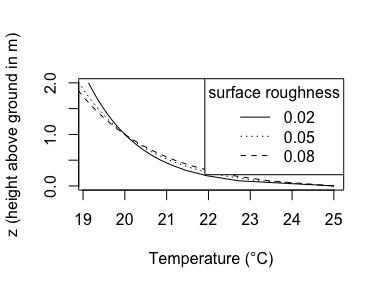Temperature at a given height is computed by the function air_temp_profile() by first estimating the temperature at roughness height:

$T_{z_0}= \frac{T_r S_{t_b}-T_0 S_{t_s}}{S_{t_b}-S_{t_s}}.$

Where $$S_{t_s}$$ is the sublayer Stanton Number $$S_{t_s}=0.62/ (\frac{z_0u^*}{12})^{0.45}$$ and $$S_{t_b}$$ is the bulk Stanton Number $$S_{t_b}=0.64/ (\frac{z}{z_0}+1)$$. The Stanton numbers are dimensionless numbers that measures the ratio of the heat transferred in and the thermal capacity. The temperature at height $$z$$ can then be estimated as:

$T_z= T_{z_0}+(T_r-T_{z_0})ln(\frac{z}{z_0}+1) .$

We estimate air temperature profiles in TrenchR as:

z.seq <- seq(0, 2, by = 0.1)

t.seq <- air_temp_profile(T_r = 20,
u_r = 0.5,
zr  = 2,
z0  = 0.02,
z   = z.seq,
T_s = 25)

plot(x    = t.seq,
y    = z.seq,
type = "l",
xlab = "Temperature (°C)",
ylab = "z (height above ground in m)")

t.seq <- air_temp_profile(T_r = 20,
u_r = 0.5,
zr  = 2,
z0  = 0.05,
z   = z.seq,
T_s = 25)

points(x    = t.seq,
y    = z.seq,
type = "l",
lty  = "dotted")

t.seq <- air_temp_profile(T_r = 20,
u_r = 0.5,
zr  = 2,
z0  = 0.08,
z   = z.seq,
T_s = 25)

points(t.seq,
z.seq,
type = "l",
lty = "dashed")

legend(x      = "topright",
title  = "surface roughness",
legend = c(0.02, 0.05, 0.08),
lty    = c("solid", "dotted", "dashed"))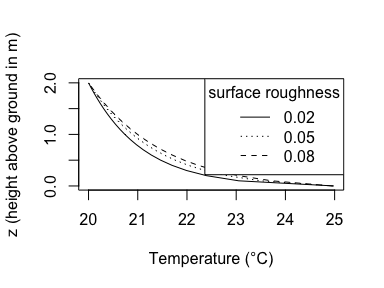TrenchR also adapts functions from NicheMapR (Kearney and Porter 2017) to refine wind speed and air temperature profiles by using multiple segments:

z.seq <- seq(0, 2, by = 0.1)
t.seq <- lapply(z.seq,
FUN = air_temp_profile_segment,
T_r = c(25, 22, 20),
u_r = c(0.01, 0.025, 0.05),
zr  = c(0.05, 0.25, 0.5),
z0  = c(0.01, 0.05, 0.1),
T_s = 27)

plot(x    = t.seq,
y    = z.seq,
type = "l",
xlab = "Temperature (°C)",
ylab = "z (height above ground in m)")

points(x = c(27, 25, 22, 20),
y = c(0, 0.05, 0.25, 0.5))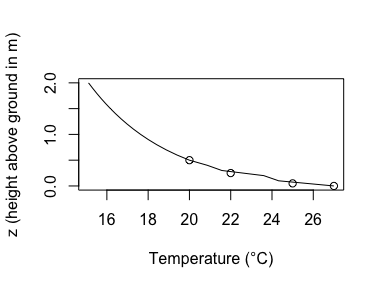z.seq <- seq(0, 2, by = 0.1)
u.seq <- lapply(z.seq,
FUN = wind_speed_profile_segment,
u_r = c(0.01, 0.025, 0.05),
zr  = c(0.05, 0.25, 0.5),
z0  = c(0.01, 0.05, 0.1))

plot(x    = u.seq,
y    = z.seq,
type = "l",
xlab = "Wind speed (m/s)",
ylab = "z (height above ground in m)",
xlim=c(0,0.05))

points(x = c(0.01, 0.025, 0.05),
y = c(0.05, 0.25, 0.5))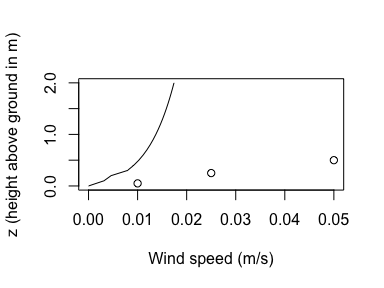# Diurnal variation in temperature and radiation

We provide three forms of equations to estimate diurnal temperature variation: a sine model, a sine exponential model, and a sine square root model. The models assume that maximum temperature $$T_x$$ will occur sometime between sunrise $$t_r$$ and sunset $$t_s$$ and that the minimum temperature $$T_n$$ will occur in the early morning.

The sine model (diurnal_temp_variation_sine()) estimates temperature $$T$$ at hour $$h$$ as a function of minimum and maximum temperature

$T(h)= T_x -T_n(1-\gamma),$

where $$\gamma=0.44-0.46 \sin (0.9+WH_r)+0.11 \sin(0.9+2WH_r)$$ and $$W=\pi/12$$.

For the sine exponential model (diurnal_temp_variation_sineexp()), hourly temperatures are estimated as a function of three empirically determined parameters: $$\alpha$$ is the time difference between the hour of maximum temperature $$h_x$$ and midday; $$\beta$$ is the time difference between the hour of minimum temperature $$h_n$$ and sunrise; and $$\gamma$$ is a decay parameter that determines the rate of temperature change from sunset to $$h'_n$$ of the next day.

The time of maximum temperature can be calculated as $$h_x=1/2(t_r+t_s)+\alpha$$. The time of minimum temperature can be calculated as $$t_n=t_r+\beta$$. The model estimates temperature at time $$h$$ as follows:

$T(h)=\left \{ \begin{array}{ll} T_n+(T_x-T_n)\sin \frac{\pi(h-h_r-\beta)}{l +2(\alpha-\beta)}, h_n \leq h \leq h_s\\ T_n'+[T(h_s)-T'_n]\exp \frac{\gamma(h-h_s)}{24-l+\beta}, h_s \leq h \leq h'_n \end{array} \right.$

where $$h'_n$$ is the time of the minimum temperature, $$T'_n$$, for the next day. Day length $$l$$ is calculated as $$h_s-h_r$$.

The sine square root model (diurnal_temp_variation_sinesqrt()) estimates temperature assuming the time of maximum temperature $$t_x$$ occurs 4 hours before sunset ($$t_x=t_s -4$$):

$T(h)=\left \{ \begin{array}{ll} T_n+\alpha \pi /2 \left \{ \frac{h-h_r}{h_x-h_r} \right \}, h_r < h \leq h_x\\ T_r+R \sin [\pi/2+(\frac{h-h_x}{4})\pi/2], h_x < h < h_s\\ T_r+b \sqrt{h-h_s}, h_s < h \leq h_p \end{array} \right.$

where $$\alpha=T_x-T_n$$,$$R=T_x-T_s$$, and $$b=\frac{T_p-T_s}{\sqrt{h_p - h_s}}$$. Sunset temperature is estimated as $$T_s=T_x-c(T_x-T_p)$$ where $$c$$ is an empirically estimated parameter ($$c=0.39$$).

TrenchR provides the functions as follows:

plot(x    = 1:23,
y    = diurnal_temp_variation_sine(T_max = 30, T_min = 10, t=1:23),
type = "l",
xlab = "hour",
ylab = "temperature (°C)")

t.seq <- lapply(1:23,
FUN   = diurnal_temp_variation_sineexp,
T_max = 30,
T_min = 10,
t_r   = 6,
t_s   = 18)

points(x    = 1:23,
y    = t.seq,
type = "l",
lty  = "dotted")

points(1:23,
y = diurnal_temp_variation_sinesqrt(t      = 1:23,
t_r    = 6,
t_s    = 18,
T_max  = 30,
T_min  = 10,
T_minp = 12),
type = "l",
lty = "dashed")

legend(x      = "topleft",
title  = "function",
legend = c("sine", "sine-exp", "sine-sqrt"),
lty    = c("solid", "dotted", "dashed"))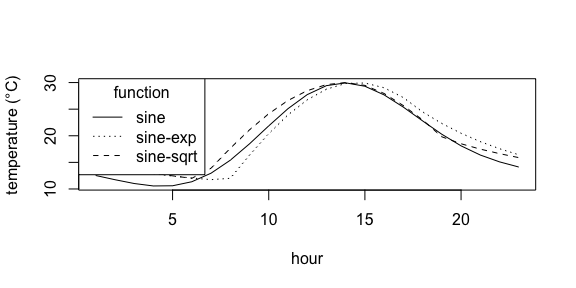We adapted equations from Tham et al. (2010) to estimate hourly solar radiation ($$W m^{-2} hr^{-1}$$) as a function of daily global solar radiation (in $$W m^{-2} d^{-1}$$). The function diurnal_radiation_variation() partitions daily solar radiation $$S$$. The function estimates the ratio of hourly to daily global irradiation,$$S_G$$, as follows:

$S_G= \frac{\pi}{24}(a'+b'\cos \omega)\frac{\cos \omega - \cos \omega_s}{\sin \omega_s - \omega_s \cos \omega_s},$

where $$a'= 0.409+0.5016\sin(\omega_s-1.047)$$ and $$b'=0.6609-0.4767\sin(\omega_s-1.047)$$.

The function utilizes two angles in radians: $$\omega$$ is the hour angle of the sun and $$\omega_s$$ is the hour angle at sunset. The function calculates these angles as a function of calendar date $$J$$ and location (latitude and longitude). We depict how the diurnal trends in radiation vary across the year (also assuming differences in total radiation).

par(mar=c(5, 5, 3, 2))

r.seq <- lapply(1:24,
doy = 172,
S = 8000,
lon = -112.07,
lat = 33.45)

plot(x    = 1:24,
y    = r.seq,
type = "l",
xlab = "hour",

r.seq <- lapply(1:24,
doy    = 356,
S      = 4000,
lon    = -112.07,
lat    = 33.45)

points(x    = 1:24,
y    = r.seq,
type = "l",
lty  = "dotted")

r.seq <- lapply(1:24,
doy    = 266,
S      = 6000,
lon    = -112.07,
lat    = 33.45)

points(x    = 1:24,
y    = r.seq,
type = "l",
lty  = "dashed")

r.seq <- lapply(1:24,
doy    = 228,
S      = 6000,
lon    = -112.07,
lat    = 33.45)

points(x    = 1:24,
y    = r.seq,
type = "l",
lty  = "dotdash")

legend(x      = "topright",
title  = "day of year",
legend = c(172, 228, 266, 356),
lty    = c("solid", "dotdash", "dashed", "dotted"))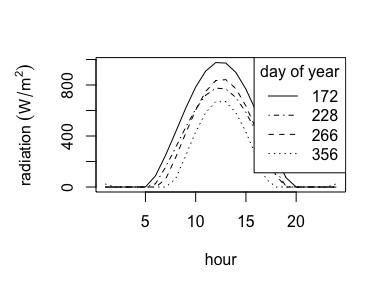# Degree days

Many organismal processes and rates are temperature dependent. Accounting for temperature dependence allows translating calendar time into a more biologically relevant concept known as degree days. Degree days indicate the accumulated heat available for processes such as development and are estimated as the accumulated product of time and temperature. For the frequent application of estimating development, degree days are calculated within physiological bounds known as the lower and upper developmental temperatures, LDT and UDT, respectively. We provide four approaches for estimating degree days that differ in the complexity with which they aggregate heat: “single.sine,” “double.sine,” “single.triangulation” or “double.triangulation.”

We implement the methods detailed here: https://ipm.ucanr.edu/WEATHER/ddfigindex.html. The methods account for whether the temperature time series is intercepted by the LDT and / or the UDT. Briefly, we present the equations for each method for the case of the temperature time series being intercepted by the lower threshold. For the single-sine method, degree days $$D$$ can be estimated as

$D= \frac{1}{\pi} \left[ \left( \frac{T_x+T_n}{2}-LDT \right) +\alpha\cos(\theta_1) \right] \\ \theta_1= \sin^{-1}\left[ 1/ \alpha \left( LDT- \frac{T_x+T_n}{2} \right) \right]$

For the double-sine method, degree days $$D$$ can be estimated as

$D= \frac{1}{2\pi} \left[ \left( \frac{T_x+T_n}{2}-LDT \right)(\pi/2- \theta_1 ) +\alpha\cos(\theta_1) \right] \\ \theta_1= \sin^{-1}\left[ 1/ \alpha \left( LDT- \frac{T_x+T_n}{2} \right) \right].$

For the single triangulation method, degree days $$D$$ can be estimated as

$D= \frac{(T_x-LDT)^2}{2(T_x-T_n)} .$

For the double triangulation method, degree days $$D$$ can be estimated as

$D= \frac{(T_x-LDT)^2}{4(T_x-T_n)}.$

The methods are available in the following R function. Note the function calculates degree days for a full day assuming symmetry in minimum temperatures.

degree_days(T_min  = 7,
T_max  = 14,
LDT    = 12,
UDT    = 33,
method = "single.sine")
##  0.47
degree_days(T_min  = 7,
T_max  = 14,
LDT    = 12,
UDT    = 33,
method = "double.sine")
##  0.47
degree_days(T_min  = 7,
T_max  = 14,
LDT    = 12,
UDT    = 33,
method = "single.triangulation")
##  0.29
degree_days(T_min  = 7,
T_max  = 14,
LDT    = 12,
UDT    = 33,
method = "double.triangulation")
##  0.29

# Soil temperatures

TrenchR includes a microclimate model that uses finite-difference methods to solve heat balance equations describing soil temperatures at the surface and specified depths. The function requires air temperature and radiation data as input. Our approach follows Porter et al. (1973) and is similar to that described for the NicheMapR R package (Kearney and Porter 2017). The primary function to call to calculate soil temperature is soil_temperature(), which is a wrapper to solve the differential equations below for equilibrium conditions. soil_temperature_function() contains the majority of the soil temperature model and calls two helper functions that implement an equation for calculating soil temperature adapted from Beckman et al. (1973): soil_temperature_integrand() includes the integrand in the equation and is called by soil_temperature_equation(), which represents the full equation.

Below we outline the soil temperature model encapsulated by the functions.

The change in soil temperature ($$T_s$$) through time $$t$$ and depth $$z$$ can be approximated with a differential equation

$\frac{dT_s}{dt}= \frac{k_s}{\rho_s c_s} \frac{\delta^2T_s}{\delta z^2}$

where $$k_s$$ is the thermal conductivity of soil (W/m·K), $$\rho_s$$ is the density of soil, and $$c_s$$ is the specific heat of soil (J/kg·K). We solve the differential equation by breaking the soil profile into depth nodes exchanging heat. We estimate the steady-state temperature profile, which is independent of initial conditions. The choice of initial conditions only affects the rate of convergence to the steady-state solution. A finite difference approximation of the differential equation yields a transient equation for the temperature at depth $$i$$ and time $$p$$ where we let $$a= \frac{k_s}{\rho_s c_s}$$:

$\frac{T_i^{p+1}-T_i^p}{dt}= a \frac{T_{i+dz}^p+T_{i-dz}^p-2T_i^p}{dz^2}.$

Solving for $$T_i^{p+1}$$ produces as expression for solving soil temperature at depth $$i$$ at any time:

$T_i^{p+1} = a \frac{dt}{dz^2}(T_{i+dz}^p+T_{i-dz}^p)+(1-2a \frac{dt}{dz^2})T_i^p$

## Soil boundary conditions

Solving the equation requires two boundary conditions: 1) the deep soil temperature, and 2) the soil surface temperature. We assume that the soil temperature at 60cm is constant diurnally. The soil surface temperature at time $$p$$ is estimated using an energy balance equating the energy conducted to the soil surface the net heat transfer to the surface by solar radiation, infrared radiation, and conduction (Beckman, Mitchell, and Porter 1973):

$Q_{net_s}= Q_{sol_s} + Q_{emit_s} + Q_{conv_s} +Q_{cond_s}$

where the solar radiation absorbed by the soil surface is the product of incoming radiation $$S$$ and the proportion of radiation absorbed $$\alpha_s$$, $$Q_{sol_s}= \alpha_s S$$.

The net thermal radiation to the soil surface is determined by the balance of radiation emitted by the soil surface $$\sigma T_s^4$$ and sky radiation absorbed by the soil surface $$\sigma T_{sky}^4$$: $$Q_{emit_s}= \epsilon_s \sigma [(T^p_{sky})^4-(T^p_0)^4]$$, where $$\epsilon_s$$ is the thermal emissivity of the soil surface and $$T^p_{sky}=0.552 T^{1.5}$$. Convection between the soil surface and air is estimated as a function of the temperature difference $$Q_{conv_s}= h_s(T^p-T^p_s)$$, where $$h_s$$ is the surface heat transfer coefficient. Consideration of temperature profiles and surface roughness yields an expression during neutral conditions (Sellers 1965):

$h_s= \frac{\rho_s c_s k^2 V_r}{ln(z_r/z_o+1)^2},$

where $$V$$ is the wind speed at reference height $$z_r$$ and $$z_0$$ is roughness length. Conduction within the soil profile is estimated as $$Q_{cond_s}=k_s \frac{\delta T_s}{\delta z}$$, at $$z=0$$. Approximating $$Q_{cond_s}$$ by finite difference yields $$Q_{cond_s}=\frac{k_s}{dz}(T^p_{dz}-T^p_s)$$, at $$z=0$$.

The net heat transfer through the profile across time is expressed as

$Q_{net_s}= \frac{\rho_s c_s dz}{2 dt} (T_0^{p+1}-T_0^p).$

Letting $$a=\frac{2dt}{\rho_s c_s dz}$$ and solving for $$T_0^{p+1}$$ in the soil energy budget yields the following boundary condition for soil surface temperature:

$T_0^{p+1}= a \alpha_s S + a \epsilon_S \sigma [(T^p_{sky})^4-(T^p_0)^4]+a h_s (T^p-T_0^p) +a \frac{k_s}{dz}(T^p_{dz}-T^p_0)+T_0^p$

Soil temperature can be estimated in TrenchR as follows. The solid line depicts air temperature (°C).

temp_vector <- diurnal_temp_variation_sine(T_max = 20,
T_min = -10,
t     = rep(1:24,4))

wind_speed_vector <- runif(n   = 96,
min = 0,
max = 0.4)

time_vector <- rep(1:24, 4)
doy    = 172,
S = 8000,
lon    = -112.07,
lat    = 33.45))

params <- list(SSA        = 0.7,
epsilon_so = 0.98,
k_so       = 0.293,
c_so       = 800,
dz         = 0.05,
z_r        = 1.5,
z0         = 0.02,
T_a        = temp_vector,
u          = wind_speed_vector,
rho_a      = 1.177,
rho_so     = 1620,
c_a        = 1006,
TimeIn     = time_vector,
dt         = 60*60,

plot(x    = 1:96,
y    = temp_vector,
type = "l",
xlab = "hour",
ylab = "temperature (°C)",
ylim = c(-10,50))

T_soil <- soil_temperature(z_r.intervals = 12,
z_r           = 1.5,
z             = 2,
T_a           = temp_vector,
u             = wind_speed_vector,
Tsoil0        = 20,
z0            = 0.02,
SSA           = 0.7,
TimeIn        = time_vector,
water_content = 0.2,
air_pressure  = 85,
rho_so        = 1620,

points(x    = 1:96,
y    = T_soil,
lty  = "dashed",
type = "l")

T_soil <- soil_temperature(z_r.intervals = 12,
z_r           = 1.5,
z             = 4,
T_a           = temp_vector,
u             = wind_speed_vector,
Tsoil0        = 20,
z0            = 0.02,
SSA           = 0.7,
TimeIn        = time_vector,
water_content = 0.2,
air_pressure  = 85,
rho_so        = 1620,

points(x    = 1:96,
y    = T_soil,
lty  = "dotted",
type = "l")

T_soil <- soil_temperature(z_r.intervals = 12,
z_r           = 1.5,
z             = 6,
T_a           = temp_vector,
u             = wind_speed_vector,
Tsoil0        = 20,
z0            = 0.02,
SSA           = 0.7,
TimeIn        = time_vector,
water_content = 0.2,
air_pressure  = 85,
rho_so        = 1620,

points(x    = 1:96,
y    = T_soil,
lty  = "dotdash",
type = "l")

legend(x      = "topright",
title  = "soil depth interval",
legend = c(2, 4, 6),
lty    = c("dashed", "dotted", "dotdash"))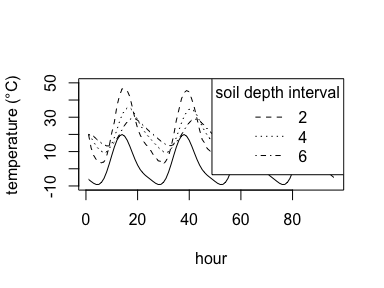## Soil specific heat and conductivity

We can approximate soil specific heat, $$c_s$$, as the weighted sum of the specific heats of soil constituents (Campbell and Norman 2012):

$\rho_s c_s= rho_m c_m x_m + rho_o c_o x_o + rho_w c_w x_w,$

where $$\rho_s$$ is the particle density of soil (bulk density), $$x$$ is the volume fraction, and the subscripts $$m$$, $$o$$,and $$w$$ stand for minerals, organic matter, and water, respectively.

We provide a function to calculate soil specific heat given the volume fractions and $$\rho_s$$:

plot(x    = seq(500,1700,100),
y    = soil_specific_heat(x_o    = 0.01,
x_m    = 0.6,
x_w    = 0.2,
rho_so = seq(500,1700,100)),
type = "l",
xlab = expression("bulk density" ~ (kg/m^{3})),
ylab = expression("soil specific heat" ~ (J ~ kg^{-1} ~ K^{-1})))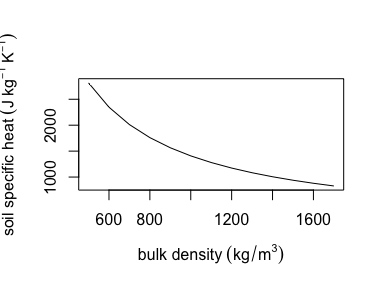suppressWarnings(par(oldpar))

Various methods of approximating soil thermal conductivity $$k_s$$ are reviewed in Boguslaw and Lukasz (2004). We provide a function soil_conductivity() to estimate $$k_s$$ using the methods of de Vries (1963). The function calculates $$k_s$$ as the weighted average of the thermal conductivities $$k$$ of soil components $$i$$:

$k_s= \frac{\sum j_i k_i x_i}{\sum j_i x_i},$

where quantity $$j_i$$ is the ratio of the average temperature gradient in the granules and the corresponding quantity in the medium (water or air):

$j_i= \frac{1}{3} \sum_{p=a,b,c}[1+\left( \frac{k_i}{k_0}-1 \right)g_{p,i}]^{-1},$

where $$k_0$$ is the conductivity of the continuous medium in which soil particles are suspended (water or air) and $$g$$ is a shape factor for the soil particles. The soil particles are assumed to be ellipsoids with axes $$g_a$$, $$g_b$$, and $$g_c$$, where $$g_a +g_b +g_c=1$$. de Vries (1952) suggests $$g_a=g_b=0.125$$. We provide the function as follows:

soil_conductivity(x      = c(0.10,0.40,0.11,0.01,0.2, 0.18),
lambda = c(0.10,0.40,0.11,0.01,0.2, 0.18),
g_a    = 0.125)
##  0.2336174